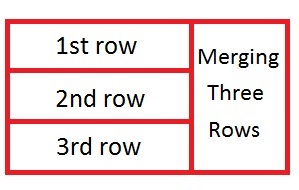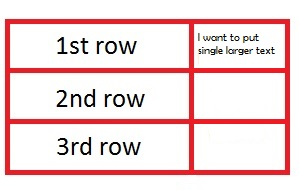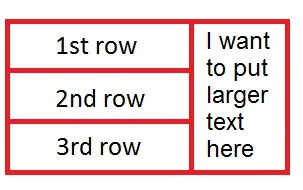# What is rowspan? How to use rowspan to merge row cells?

## What is rowspan

A rowspan is the attribute used in table header cells and data cells to merge rows. Merging is the same feature we use Microsoft Excel. In some cases, We need to combine two or more than two rows. We use rowspan in these cases.## How we use rowspan in tables

To explain this how we use rowspan, Let us take a simple example:

Suppose I want to use table contains 3 rows and two columns. In the first column of the table, I want to include data to each row one by one. I put data to the 1st row of 1st column, 2nd row of 1st column, 3rd row of the 1st column. Now in the 2nd column, I want to put only a single line larger length data. This data should not look ugly at the table.

Here, If we put single data in the 2nd column without merging the rows. We put it on the 1st and in the 2nd column of the table like this:But if we do this and put the data in the table. The two cells remain vacant in the table. It looks like the table is not arranged in the managed way.

Apply this example using HTML code:

Output

 PHP These are the web development codes on combining gives a Web page. CSS HTML

We need to merge the three rows to display the data in the managed way. In this manner, I merge the three rows using colspan attribute of the table. We need to use ‘rowspan = 3’ to merge the 3 rows of the table. After I merge the rows the table will appear like this:Here, In this image, we can see that, I have merged the three rows and put the text with the larger font size.

Let us see this with ‘rowspan’ attribute using in the HTML code:

Output

 PHP These are the web development codes on combining gives a Web page. CSS HTML

If we want to merge the two rows of a table, we use rowspan = “2”.

Similarly, if we want to merge three rows of a table, we use rowspan=”3″ and so on.